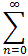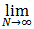#Interactive Real Analysis

Next | Previous | Glossary | Map

## 8.3. Series and Power Series

### Definition 8.3.1: Function Series

Suppose { fn(x) } is a sequence of functions and we define the N-th partial sum as
SN(x) =fn(x)
Let D be the set of points for which the sequence of partial sums converges pointwise. Then, for xD, we denote the resulting limit function byfn(x) =SN(x) =fn(x)

Next | Previous | Glossary | Map